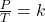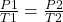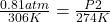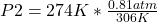## A bottle containing air is initially at a temperature of 33 degrees C and a pressure of 0.81 atm. After being placed in the freezer, the fin

Question

A bottle containing air is initially at a temperature of 33 degrees C and a pressure of 0.81 atm. After being placed in the freezer, the final temperature is 1 degrees C. What is the final pressure ?

in progress 0
4 months 2021-09-05T13:25:29+00:00 1 Answers 4 views 0

## Answers ( )

The final pressure is 0.725 atm.

Explanation:

Gay Lussac’s Law establishes the relationship between pressure and temperature of a gas when the volume is constant. This law says that when there is a constant volume, as the temperature increases, the pressure of the gas increases. And when the temperature is decreased, the pressure of the gas decreases. That is, pressure and temperature are directly proportional quantities.

Mathematically, Gay-Lussac’s law states that, when a gas undergoes a constant volume transformation, the quotient of the pressure exerted by the gas temperature remains constant:When analyzing an initial state 1 and a final state 2, the following is satisfied:In this case:

• P1= 0.81 atm
• T1= 33 C= 306 K
• P2= ?
• T2= 1 C= 274 K

Replacing:Solving:P2= 0.725 atm

The final pressure is 0.725 atm.